AB and CD are two straight very long parallel conductors placed in air at a distance a. They carry currents I1 and I2 respectively.(Fig 3.23) The magnetic induction due to current I1 in AB at a distance a is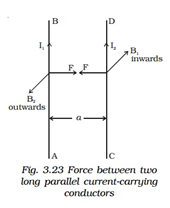This magnetic field acts perpendicular to the plane of the paper and inwards. The conductor CD with current I2 is situated in this magnetic field. Hence, force on a segment of length l of CD due to magnetic field B1 isSubstituting equation (1)By Fleming’s Left Hand Rule, F acts towards left. Similarly, the magnetic induction due to current I2 flowing in CD at a distance a is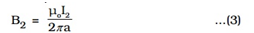This magnetic field acts perpendicular to the plane of the paper and outwards. The conductor AB with current I1, is situated in this field. Hence force on a segment of length l of AB due to magnetic field B2 is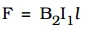Substituting equation (3)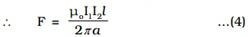By Fleming’s left hand rule, this force acts towards right. These two forces given in equations (2) and (4) attract each other. Hence, two parallel wires carrying currents in the same direction attract each other and if they carry currents in the opposite direction, repel each other.

### Definition of ampere

The force between two parallel wires carrying currents on a segment of length l is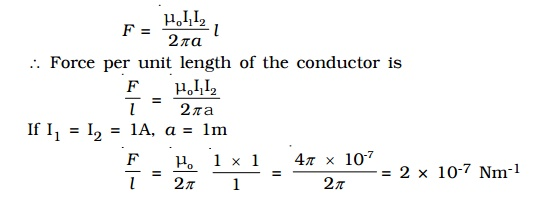The above conditions lead the following definition of ampere.

Ampere is defined as that constant current which when flowing through two parallel infinitely long straight conductors of negligible cross section and placed in air or vacuum at a distance of one meter apart, experience a force of 2 × 10-7 Newton per unit length of the conductor.